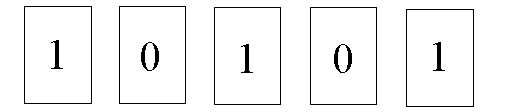# Just Zeros and Ones

Grade Level: Primary School, Middle School, and High School
Math Investigation Type: Numbers

#### LEVEL:

This investigation can be entered into by children as young as 6 (particularly as it is structured in the game situation) and is entertaining for people who like to think about different number bases and find mathematical patterns. Playing with different number bases leads nicely into thinking about factoring and logarithms. The reason information theory makes such frequent use of logarithms base two is that the most fundamental unit of information is a bit, and a bit of information represents a choice between two possibilities.

#### MATERIALS:

Cards with 1, 2, 4, 8 and 16 dots (more for higher grades, following the same pattern)
Cards labelled 0 and 1

#### OBJECTIVE:

to extend an understanding of place value
to introduce another number base
to introduce a bases for computer technology

Number systems, number concepts, patterns, number representations, simulation, mathematical processes, numerical computation, visualization.

1. Divide the group into two teams.
2. The first team picks a number less than 32 (higher grades can go greater than this) and represents it using the dotted cards. For example: say the team chooses the number 21. They would use the dotted cards with 1, 4 and 16 (each card being used at most once).
3.The second team must use this binary representation to reconstruct the number using their dotted cards.
4. The second team picks a number less than 32 (higher grades can go greater than this) and represents it using the dotted cards. The second team now converts these dotted cards to their binary representation using the 0 and 1 cards and sends the first team and the first team must reconstruct the number using their dotted cards.
5. The play goes back to the first team.

### By The Way of Explanation

When we write our numbers we use the digits 0,1,2,3,4,5,6,7,8,9. This is called base ten because we use ten digits to form any number. The placement of the digit in the number stands for that digit times a power of ten. When we write our numbers, each digit’s value depends on its place in the number

For example: 5374 does not mean 5 + 3 + 7 + 4, but rather

5 thousands + 3 hundreds + 7 tens + 4 ones

Each “place” in the number is a power of ten:

thousand = 1000 = 10 x 10 x 10 x 10 = 103

hundred = 100 = 10 x 10 = 102

ten = 10 = 101

one = 1 = 100

Computers write their numbers using only the digits 0 and 1. Their system is called base two because only the first two digits are used to form numbers and each place in the number corresponds to a power of two. The first place is the 1’s place, then comes the 2’s place, then the 2 x 2 = 4’s place, then the 2 x 2 x 2 x 2 = 16’s place, and so on.

 Base Ten Base Two Base Ten Base Two Base Ten Base Two Base Ten Base Two 0 0 10 1010 20 10100 30 11110 1 1 11 1011 21 10101 31 11111 2 10 12 1100 22 10110 32 100000 3 11 13 1101 23 10111 4 100 14 1110 24 11000 5 101 15 1111 25 11001 6 110 16 10000 26 11010 7 111 17 10001 27 11011 8 1000 18 10010 28 11100 9 1001 19 10011 29 11101

### Questions

Can every number smaller than 32 be represented this way?
Are there different ways to represent a number?
What about 32 and higher cards?

### Extension

Design a new game by switching base 2 to any other base. In base 5 for example, you will need dotted cards with 1, 5, 25, 125 dots, and cards labelled 0, 1, 2, 3, and 4. Right? In representing a number you might use the card with 25 dots for example up to 4 times.
You may also want to look for the game of “NIM.”

### Applications

Computer operations are performed using this simple number system because transistor (switch) mechanics have two basic conditions, on and off. On is equal to “one” while off is equal to “zero.” In the microprocessor, electrical pulses turn transistors on or off. Strings of on’s and off’s can be translated in this way into a mathematical language called binary code.

Base ten works very well with our pen and pencil calculations, but another base system is needed for electronic computers. If a memory device were to operate in base ten it would have to assume ten different states for the ten numerals comprising base ten. Although this is possible with a mechanical system, it is not feasible with electricity. On the other hand the binary base system is a perfect candidate for electronic computers. These two numerals can be easily represented by electricity in one of three ways:

• by having the current either on or off
• by magnetizing a coil in one direction or the other
• by energizing (or not energizing) a relay